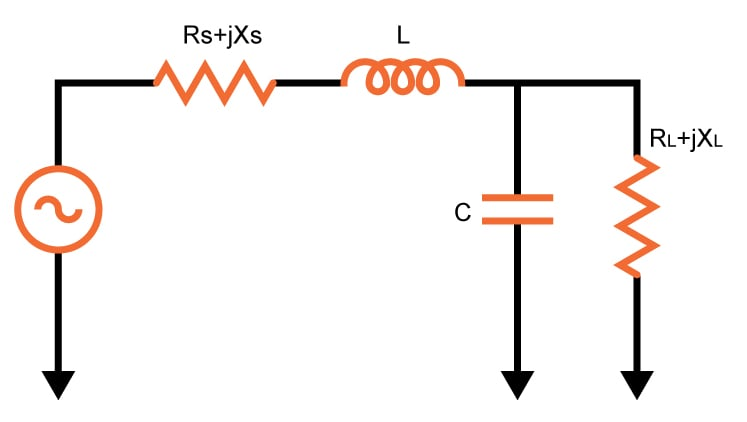# L-Match Impedance Matching Circuits

## This calculator is designed to compute for the parameters needed to design a L-Match Impedance Circuit

Ohm
Ohm
Ohm
Ohm

### Overview

The L-match impedance matching circuit is one of the circuits used to match the impedance between two points, usually a source and a load. The circuit got its name because the inductor and the capacitor form an L-shape (see schematic below). Note that the inductor and capacitor can be interchanged depending on the input. If you need to block direct current, then the capacitor is placed near the source. Otherwise, the inductor is placed near the source, as seen in the schematic below.This calculator will help you determine the correct values for the inductor and capacitor in an L-match impedance matching circuit. The required parameters are the signal (or source) frequency, the impedance of the source and the impedance of the load. You can also specify if the circuit will pass direct current or block it. The inductor quality factor is also computed.

### Equations

For Pass DC Current:

$$Z_{input}=\left [ \left \{ R_{L}+jX_{L}+\left ( \frac{1}{j\cdot \omega\cdot C} \right )\right \}//\left ( j\omega\cdot L \right ) \right]$$

For Block DC Current:

$$Z_{input}=\left \{\left [R_{L}+jX_{L}+ ( j\omega\cdot L ) \right ]// \left ( \frac{1}{j\cdot \omega\cdot C } \right ) \right \}$$

### Applications

Impedance matching is important in electrical engineering because it allows for maximum power transfer between two points. In telephone systems, for example, minimizing echo on long-distance lines is achieved using match impedances. The telephone hybrid coil, where two wires are converted into four wires can also be achieved via matching.

In audio amplifiers, impedances are not matched as it is typical for amplifiers to have output impedances that are lower than the load impedance for better speaker damping. But for higher-power amplifiers, such as those that use vacuum tubes, impedance-changing circuits are used to get a low output impedance in order to better match the amplifier's performance to the load impedance.